Simple hypothesis testing

A t-test is any statistical hypothesis test in which the test statistic follows a Student's t-distribution if the null hypothesis is supported. It can be used to determine if two sets of data are significantly different from each other, and is most commonly applied when the test statistic would follow a normal distribution if the value of a scaling term in the test statistic were known.

?TDist

For those who have never looked at the Students t distribution of values, we can plot a sample:

plot( dt(-10:10, 1) )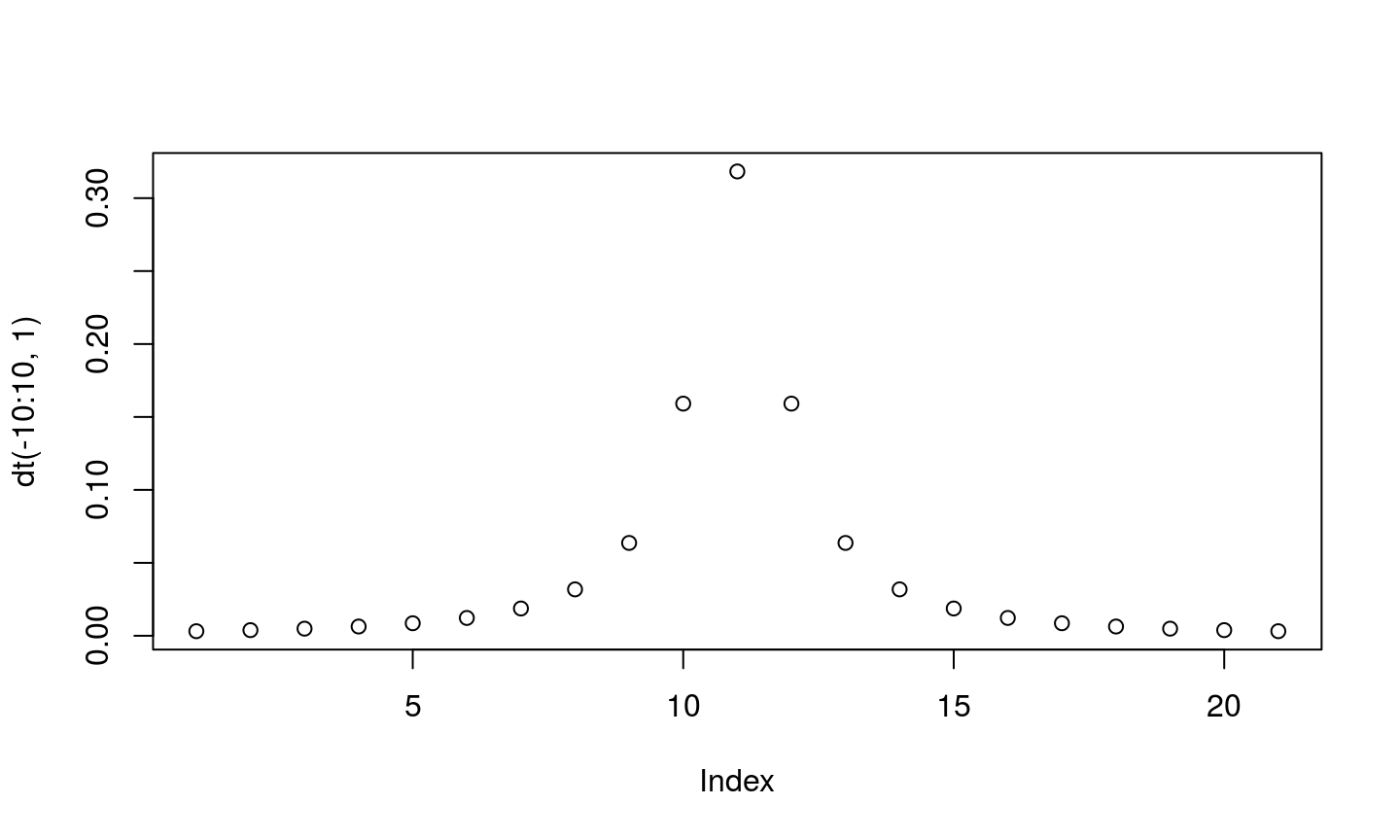Analysis of variance (ANOVA) is a collection of statistical models used to analyze the differences among group means and their associated procedures (such as "variation" among and between groups), developed by statistician and evolutionary biologist Ronald Fisher.

Fire up the ANOVA Playground app and we'll talk about how these tests work.

Expressing hypotheses with formulas

A ~ B

Average task time (ObjectAve) is a function of task complexity (Complexity)

We could use this formula syntax in a call to t.test:

t.test(ObjectAve ~ Complexity, data = jdTidy)
##
##  Welch Two Sample t-test
##
## data:  ObjectAve by Complexity
## t = -4.4255, df = 337.69, p-value = 1.3e-05
## alternative hypothesis: true difference in means is not equal to 0
## 95 percent confidence interval:
##  -1.0053871 -0.3866584
## sample estimates:
## mean in group complex  mean in group simple
##              4.397727              5.093750
boxplot(ObjectAve ~ Complexity, data = jdTidy)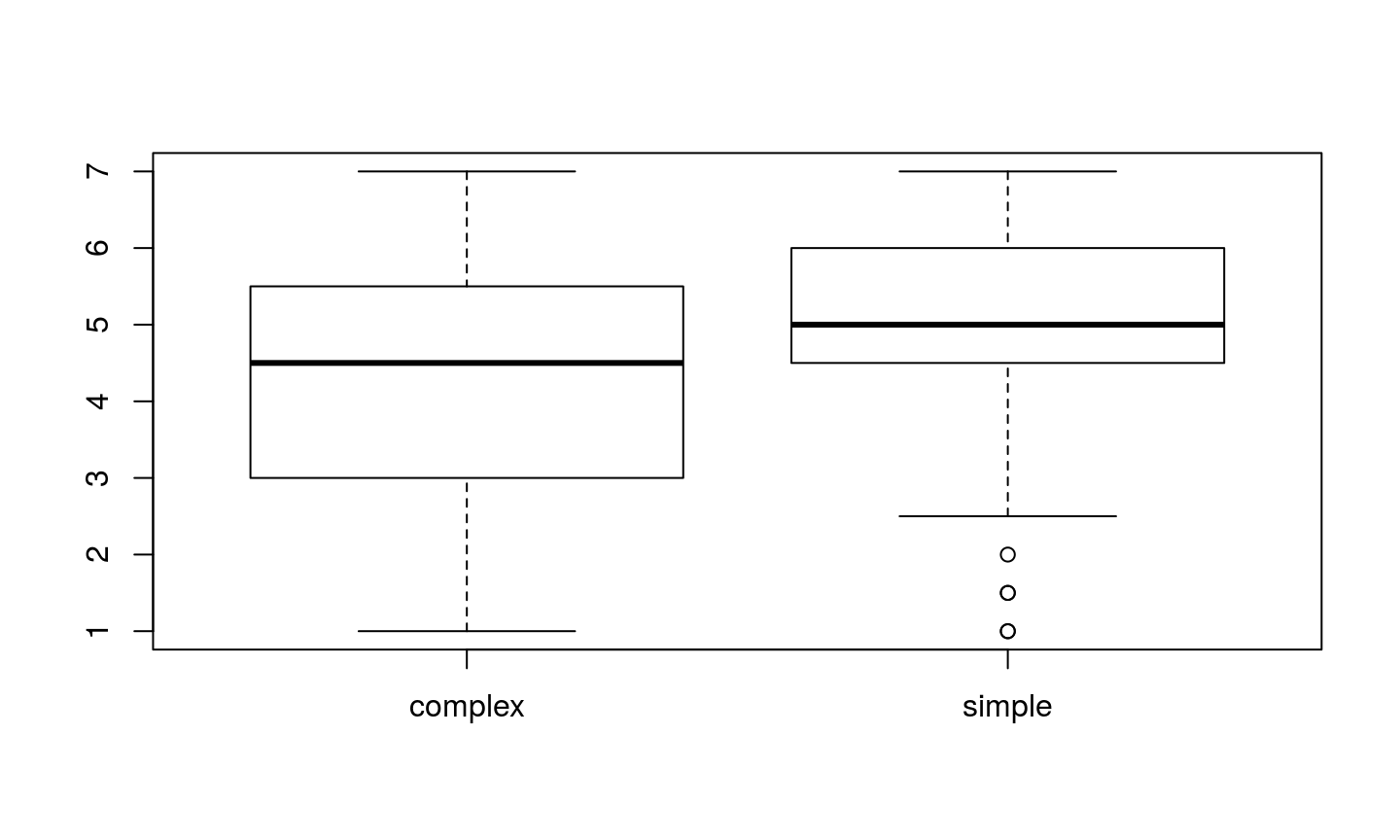test <- aov(ObjectAve ~ Complexity, data = jdTidy)
summary(test)
##              Df Sum Sq Mean Sq F value   Pr(>F)
## Complexity    1   42.6   42.63   19.59 1.29e-05 ***
## Residuals   350  761.9    2.18
## ---
## Signif. codes:  0 '***' 0.001 '**' 0.01 '*' 0.05 '.' 0.1 ' ' 1
plot(test)

The plot function is magic!

summary(aov(ObjectAve ~ Education, data = jdTidy))
##              Df Sum Sq Mean Sq F value Pr(>F)
## Education     6   13.6   2.264   0.994  0.429
## Residuals   343  780.7   2.276
## 2 observations deleted due to missingness
boxplot(ObjectAve ~ Education, data = jdTidy)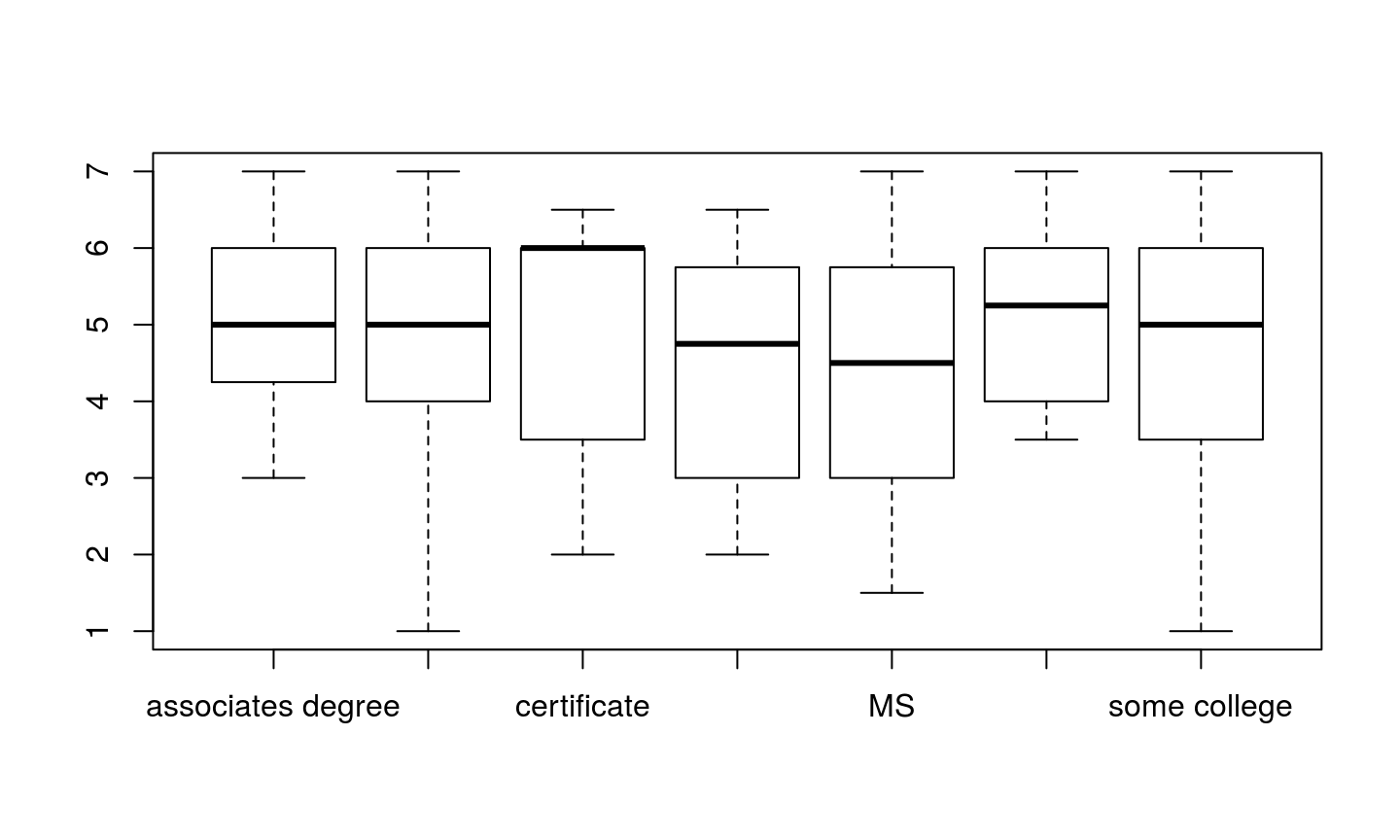summary(aov(ObjectAve ~ Complexity + Gender, data = jdTidy))
##              Df Sum Sq Mean Sq F value   Pr(>F)
## Complexity    1   42.6   42.63  19.953 1.07e-05 ***
## Gender        1   16.2   16.20   7.582   0.0062 **
## Residuals   349  745.7    2.14
## ---
## Signif. codes:  0 '***' 0.001 '**' 0.01 '*' 0.05 '.' 0.1 ' ' 1
summary(aov(ObjectAve ~ Complexity:Gender, data = jdTidy))
##                    Df Sum Sq Mean Sq F value   Pr(>F)
## Complexity:Gender   3   62.7  20.903   9.807 3.17e-06 ***
## Residuals         348  741.8   2.132
## ---
## Signif. codes:  0 '***' 0.001 '**' 0.01 '*' 0.05 '.' 0.1 ' ' 1

Here's the same along side the previous models:

summary(aov(ObjectAve ~ Complexity + Gender + Complexity:Gender, data = jdTidy))
##                    Df Sum Sq Mean Sq F value   Pr(>F)
## Complexity          1   42.6   42.63   20.00 1.05e-05 ***
## Gender              1   16.2   16.20    7.60  0.00615 **
## Complexity:Gender   1    3.9    3.88    1.82  0.17821
## Residuals         348  741.8    2.13
## ---
## Signif. codes:  0 '***' 0.001 '**' 0.01 '*' 0.05 '.' 0.1 ' ' 1

Because this is a common formulation, you can use a * to do the same:

summary(aov(ObjectAve ~ Complexity * Gender, data = jdTidy))
##                    Df Sum Sq Mean Sq F value   Pr(>F)
## Complexity          1   42.6   42.63   20.00 1.05e-05 ***
## Gender              1   16.2   16.20    7.60  0.00615 **
## Complexity:Gender   1    3.9    3.88    1.82  0.17821
## Residuals         348  741.8    2.13
## ---
## Signif. codes:  0 '***' 0.001 '**' 0.01 '*' 0.05 '.' 0.1 ' ' 1

Let's look at a picture to see what's going on there:

boxplot(ObjectAve ~ Complexity * Gender, data = jdTidy)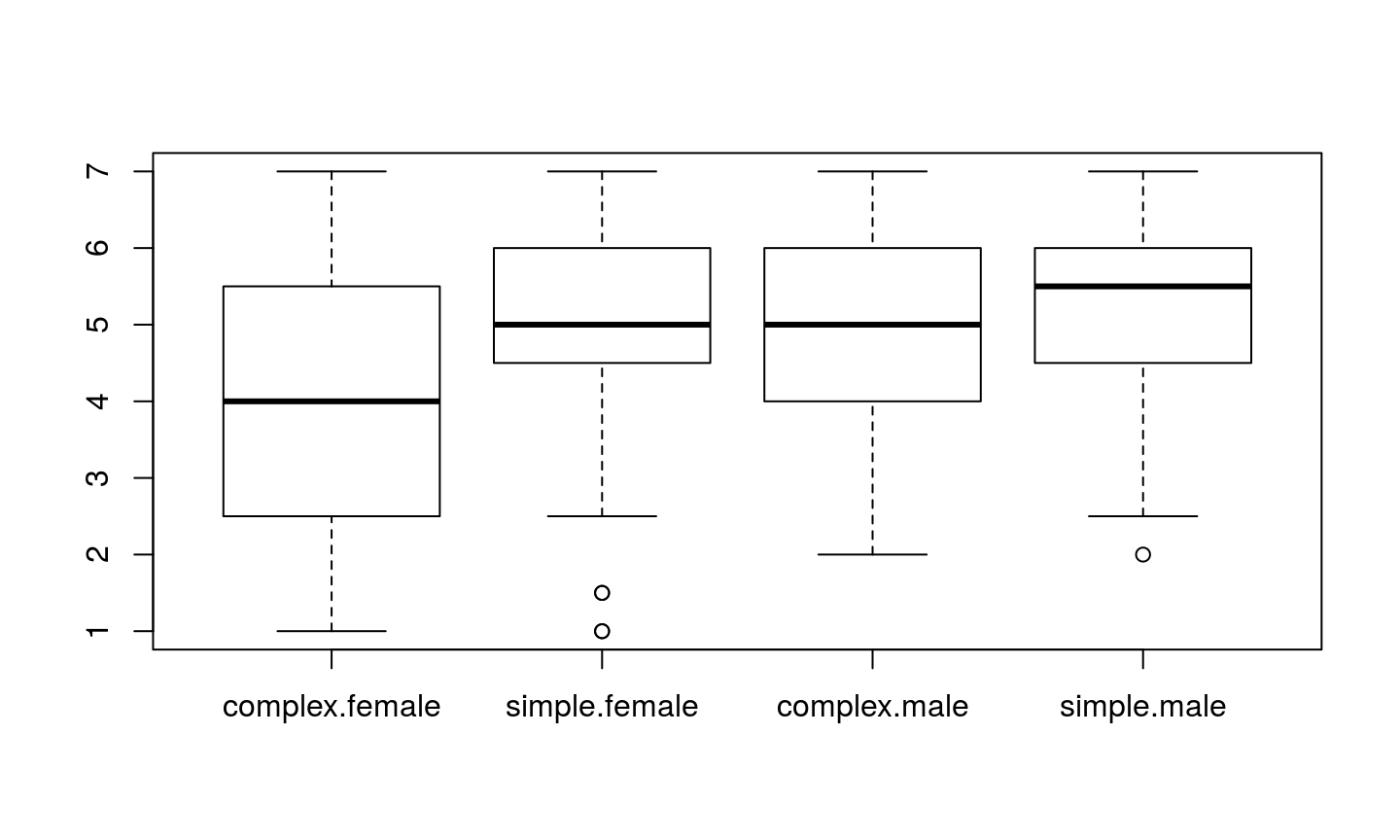See the help page for formula for additional syntax that can be used in formula expressions.

# Enter your code here!

Regression

plot(VelcroTime ~ VacuumTime, data = jdFact)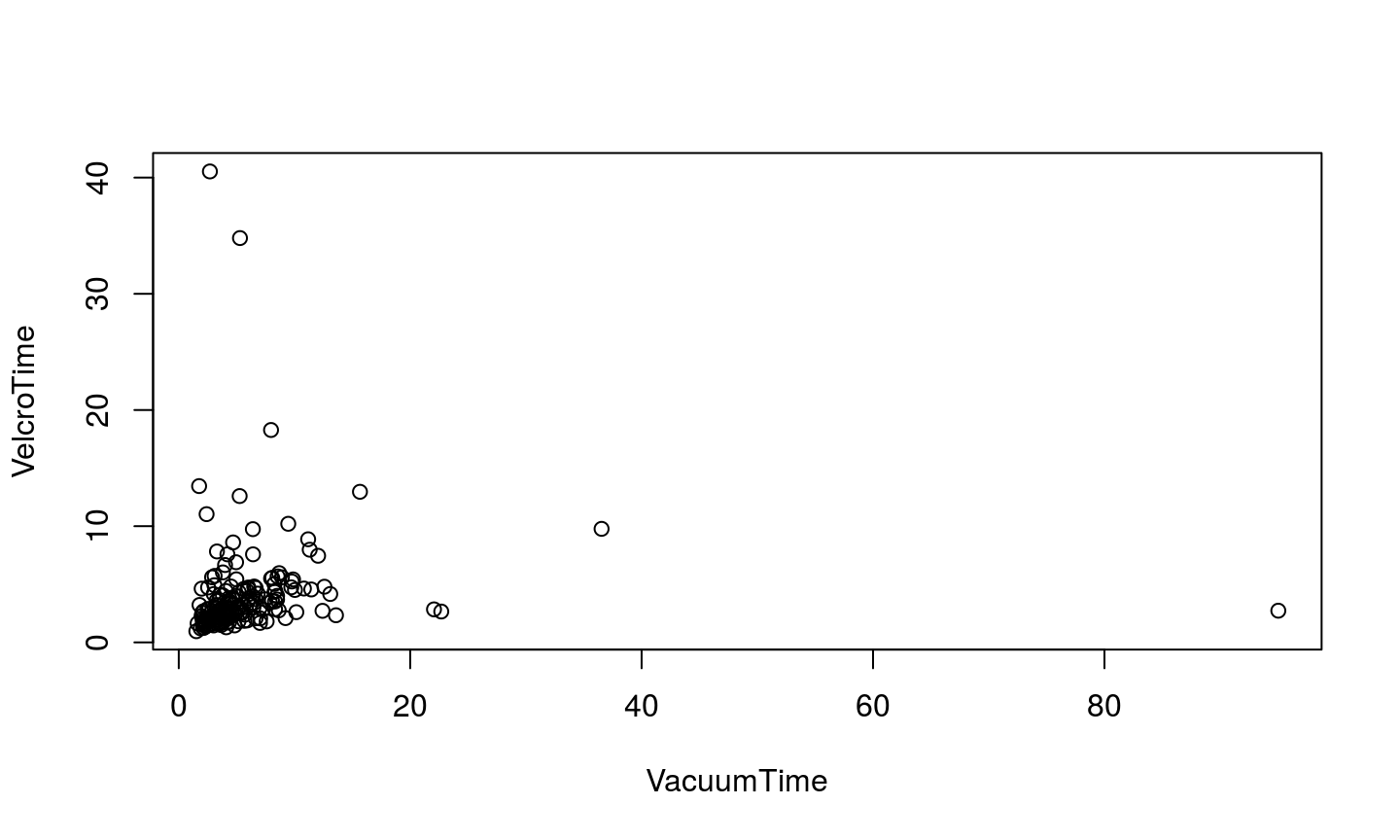fit <- lm(VelcroTime ~ VacuumTime, data = jdFact)
fit
##
## Call:
## lm(formula = VelcroTime ~ VacuumTime, data = jdFact)
##
## Coefficients:
## (Intercept)   VacuumTime
##     3.88202      0.03299
cf <- coefficients(fit)
cf
## (Intercept)  VacuumTime
##  3.88201636  0.03298668
summary(fit)
##
## Call:
## lm(formula = VelcroTime ~ VacuumTime, data = jdFact)
##
## Residuals:
##    Min     1Q Median     3Q    Max
## -4.287 -1.931 -1.090  0.359 36.561
##
## Coefficients:
##             Estimate Std. Error t value Pr(>|t|)
## (Intercept)  3.88202    0.42088   9.224   <2e-16 ***
## VacuumTime   0.03299    0.04239   0.778    0.437
## ---
## Signif. codes:  0 '***' 0.001 '**' 0.01 '*' 0.05 '.' 0.1 ' ' 1
##
## Residual standard error: 4.409 on 174 degrees of freedom
## Multiple R-squared:  0.003469,   Adjusted R-squared:  -0.002258
## F-statistic: 0.6057 on 1 and 174 DF,  p-value: 0.4375

We can plot the fitted model on top of our scatter plot using abline:

plot(VelcroTime ~ VacuumTime, data = jdFact)
abline(reg = fit)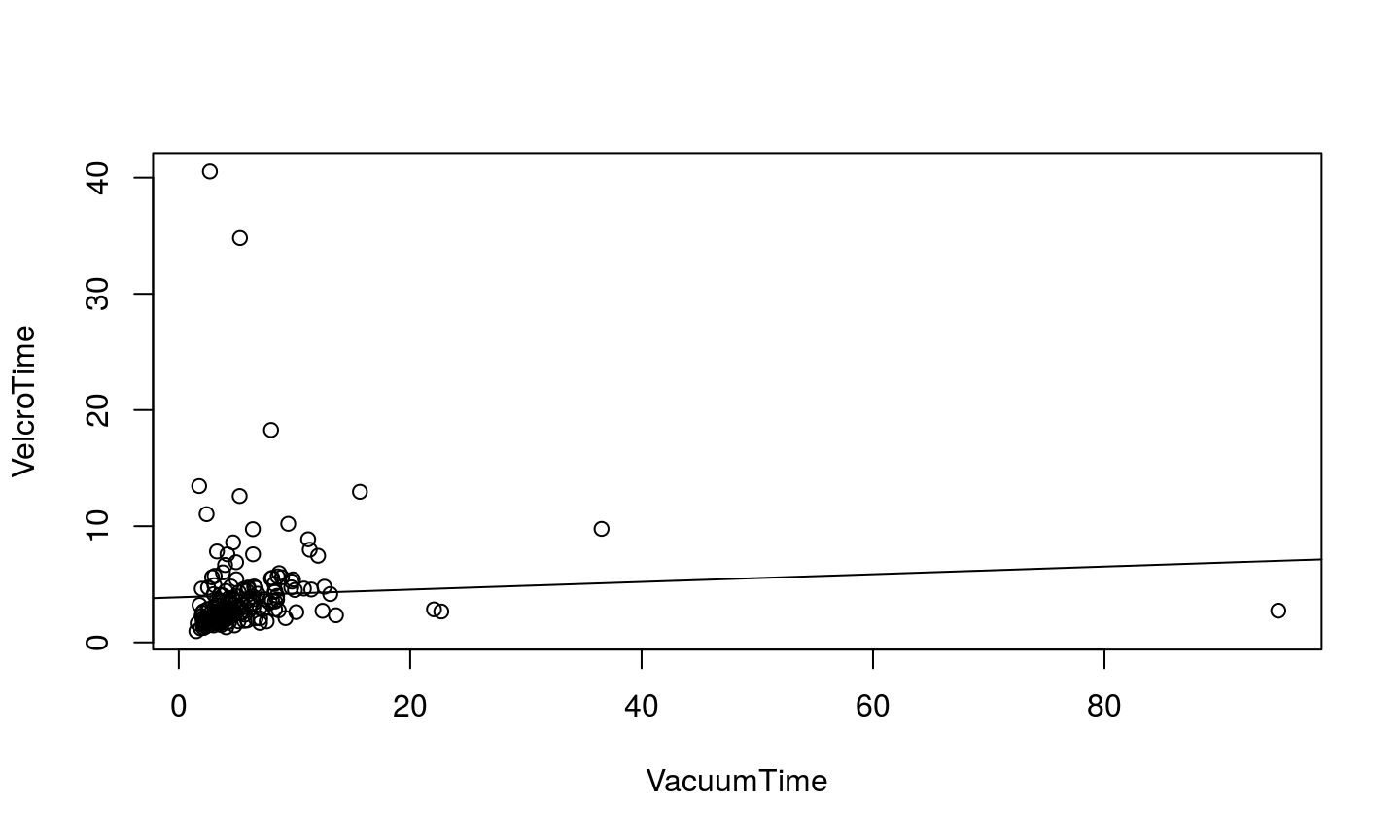lm(VelcroTime ~ log(VacuumTime), data = jdFact)
##
## Call:
## lm(formula = VelcroTime ~ log(VacuumTime), data = jdFact)
##
## Coefficients:
##     (Intercept)  log(VacuumTime)
##           2.513            1.002

After class

• Read Goffeau et al. 1996
• Make sure you have a working solution to the translation problem at the end of Working with Tables exercise (#2).